# A charged particle moving through a magnetic field at right angles to the field with a...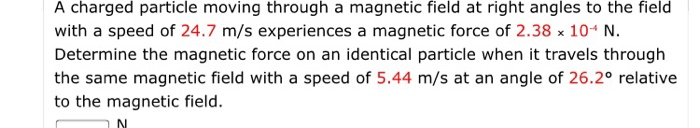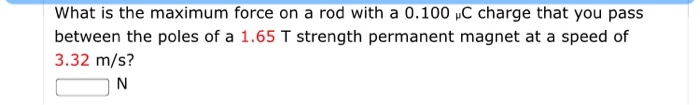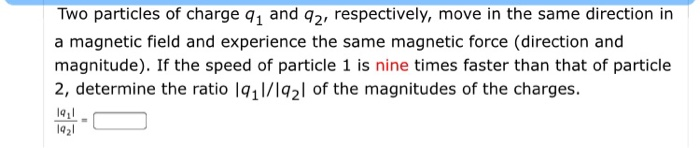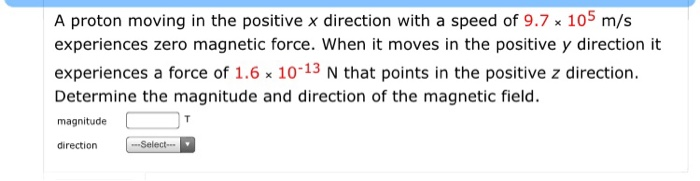A charged particle moving through a magnetic field at right angles to the field with a speed of 24.7 m/s experiences a magnetic force of 2.38 x 10* N. Determine the magnetic force on an identical particle when it travels through the same magnetic field with a speed of 5.44 m/s at an angle of 26.2° relative to the magnetic field. N
What is the maximum force on a rod with a 0.100 C charge that you pass between the poles of a 1.65 T strength permanent magnet at a speed of 3.32 m/s? N
Two particles of charge 91 and 92, respectively, move in the same direction in a magnetic field and experience the same magnetic force (direction and magnitude). If the speed of particle 1 is nine times faster than that of particle 2, determine the ratio 1911/1921 of the magnitudes of the charges. 19,1 1921
A proton moving in the positive x direction with a speed of 9.7 x 105 m/s experiences zero magnetic force. When it moves in the positive y direction it experiences a force of 1.6 x 10-13 N that points in the positive z direction. Determine the magnitude and direction of the magnetic field. magnitude direction --Select-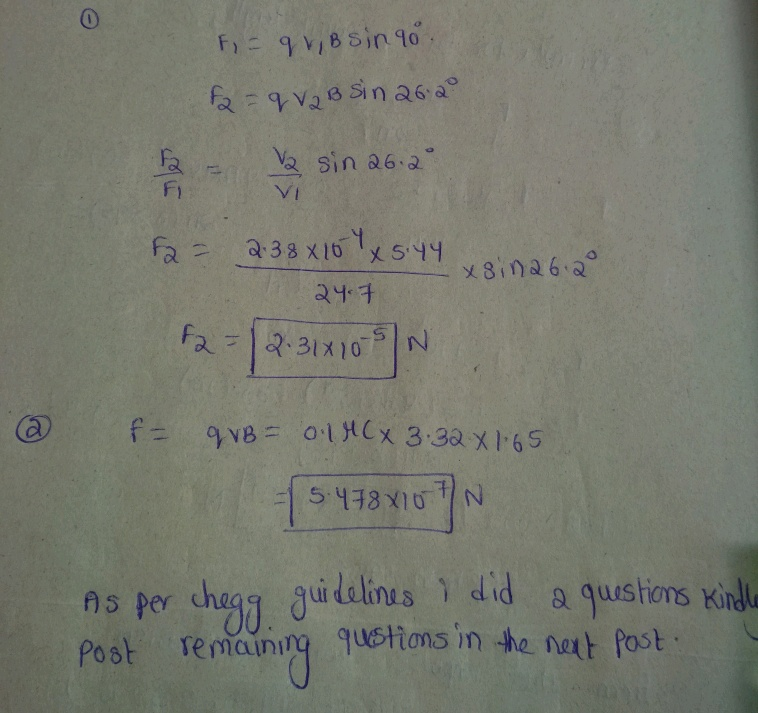##### Add Answer of: A charged particle moving through a magnetic field at right angles to the field with a...
Similar Homework Help Questions
• ### A charged particle moving through a magnetic field at night angles to the field with a...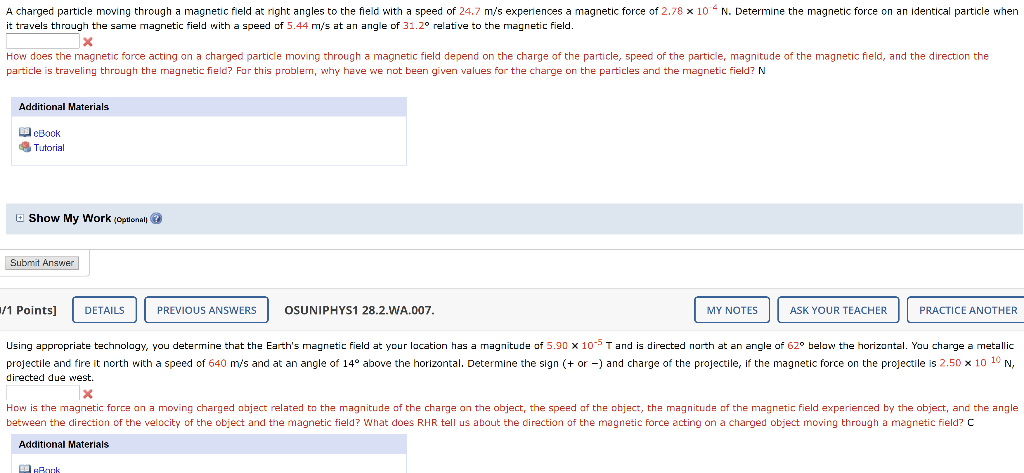A charged particle moving through a magnetic field at night angles to the field with a speed of 24.7 m/s experiences a magnetic force of 2.78 x 10 N. Determine the magnetic force on an identical particle when it travels through the same magnetic field with a speed of 5.44 m/s at an angle of 31.20 relative to the magnetic field. x How does the magnetic force acting on a charged particle moving through a magnetic field depend on the...

• ### Particle moving through a Magnetic Field!

A particle with a charge of 13 mu C experiences a force of 2.2×10-4 N when it moves at right angles to a magnetic field with a speed of 26 m/s.What force does thisparticle experience when it moves with a speed of 6.4 m/s at an angle of 27^circ relative to the magnetic field?

• ### The force on a charged particle moving in a magnetic field can be computed as the...

The force on a charged particle moving in a magnetic field can be computed as the vector sum of the forces due to each separate component of the magnetic field. As an example, a particle with charge q is moving with speed v in the? y-direction. It is moving in a uniform magnetic field Part A What is the x-component of the force F?  exerted on the particle by the magnetic field? Part B What is the y-component of the force...

• ### A charged particle is moving at an angle of 60 in a magnetic field of magnitude...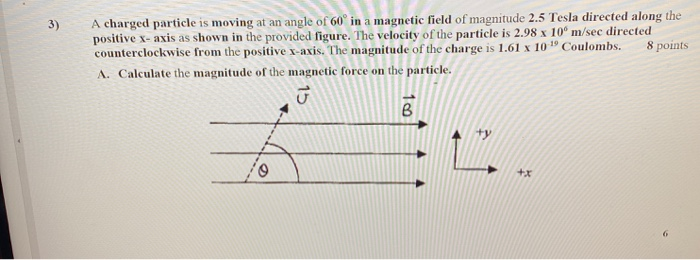A charged particle is moving at an angle of 60 in a magnetic field of magnitude 2.5 Tesla directed along the positive X-axis as shown in the provided figure. The velocity of the particle is 2.98 x 10 m/sec directed counterclockwise from the positive x-axis. The magnitude of the charge is 1.61 x 10 "Coulombs. 8 points A. Calculate the magnitude of the magnetic force on the particle.

• ### An electron moving at 19489423 m/s in the positive x-direction at right angles to a magnetic...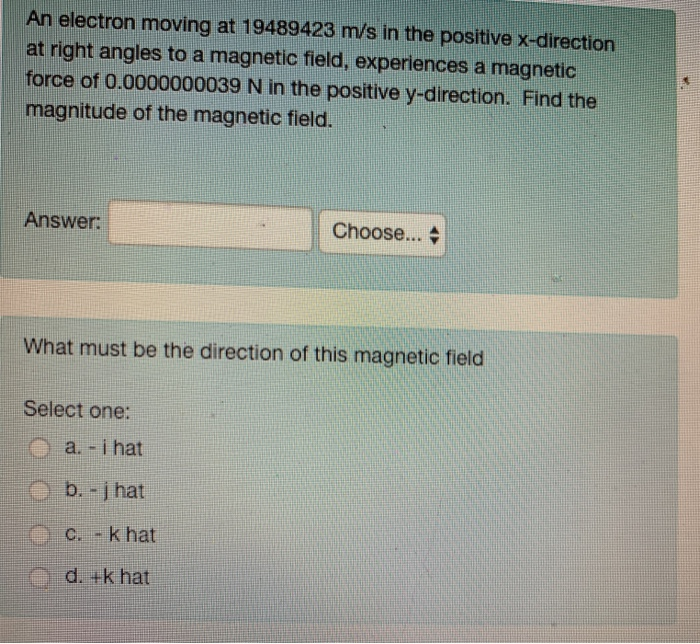An electron moving at 19489423 m/s in the positive x-direction at right angles to a magnetic field, experiences a magnetic force of 0.0000000039 N in the positive y-direction. Find the magnitude of the magnetic field Answer: | Choose ▲ What must be the direction of this magnetic field Select one: a. -i hat d. +k hat

• ### 3) A charged particle is moving with velocity of V in a magnetic field of B,...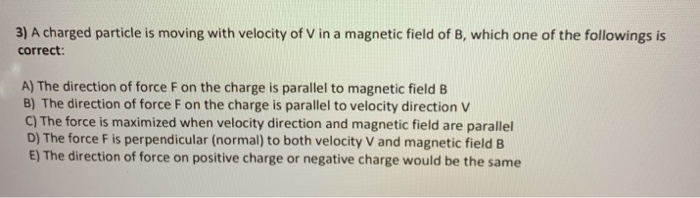3) A charged particle is moving with velocity of V in a magnetic field of B, which one of the followings is correct: A) The direction of force F on the charge is parallel to magnetic field B B) The direction of force F on the charge is parallel to velocity direction V C) The force is maximized when velocity direction and magnetic field are parallel D) The force F is perpendicular (normal) to both velocity V and magnetic field...

• ### Particle in the magnetic field

A particle having an electric charge q = 3.20×10-19 C is injected into a magnetic field B = 0.24 T with a speed of 1.57×107 m/s. The velocity of the particle isperpendicular to the direction of the magnetic field.What is a magnitude of the magnetic force on the particle?How great an electric field would be required to exert an electrostatic force with the same magnitude as the magnetic force acting on the particle? ( V/m)

• ### Particle in the magnetic field

A particle having an electric charge q = 3.20×10-19 C is injected into a magnetic field B = 0.14 T with a speed of 1.00×107 m/s. The velocityof the particle is perpendicular to the direction of the magnetic field.Part 1:What is a magnitude of the magnetic force on the particle?Part 2:How great an electric field would be required to exert an electrostatic force with the same magnitude as the magnetic force acting on the particle? (V/m)

• ### magnetic forces on moving charge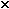An electron moving with a speed of 8.4105 m/s in the positive z directionexperiences zero magnetic force.When it moves in the positive x direction it experiences a force of2.0 10-13 N that points in the negative y direction. What is the magnitude anddirection of the magnetic field?

• ### An electron moving at 19817425 m/s in the positive x-direction at right angles to a magnetic...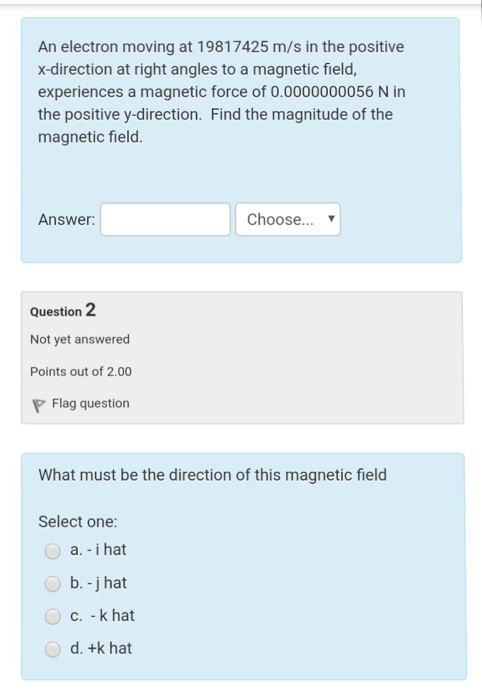An electron moving at 19817425 m/s in the positive x-direction at right angles to a magnetic field, experiences a magnetic force of 0.0000000056 N in the positive y-direction. Find the magnitude of the magnetic field. Answer Choose... Question 2 Not yet answered Points out of 2.00 Flag question What must be the direction of this magnetic field Select one: a. - i hat b. -j hat c. - k hat d. +k hat

Free Homework App